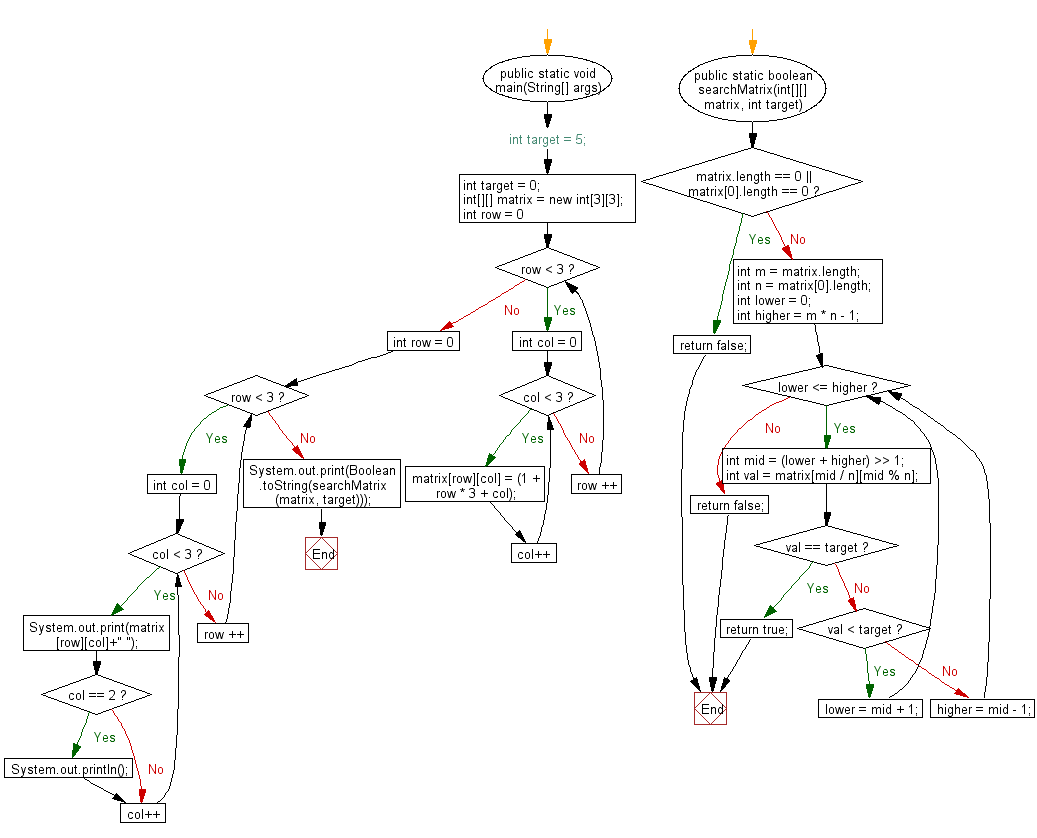﻿ Java exercises: Searches a value in an m x n matrix - w3resource# Java Exercises: Searches a value in an m x n matrix

## Java Basic: Exercise-120 with Solution

Write a Java program that searches a value in an m x n matrix.

Sample Solution:

Java Code:

``````public class Main {
public static void main(String[] args) {
// int target = 5;
int target = 0;
int[][] matrix = new int;
for (int row = 0; row < 3; row ++)
for (int col = 0; col < 3; col++)
matrix[row][col] = (1 + row * 3 + col);

for (int row = 0; row < 3; row ++)
{
for (int col = 0; col < 3; col++)
{
System.out.print(matrix[row][col]+" ");
if (col == 2)
System.out.println();
}
}
System.out.print(Boolean.toString(searchMatrix(matrix, target)));
}
public static boolean searchMatrix(int[][] matrix, int target) {
if (matrix.length == 0 || matrix.length == 0) {
return false;
}
int m = matrix.length;
int n = matrix.length;
int lower = 0;
int higher = m * n - 1;
while (lower <= higher) {
int mid = (lower + higher) >> 1;
int val = matrix[mid / n][mid % n];
if (val == target) {
return true;
}
if (val < target) {
lower = mid + 1;
} else {
higher = mid - 1;
}
}
return false;
}
}

```
```

Sample Output:

```1 2 3
4 5 6
7 8 9
false
```

Pictorial Presentation:Flowchart:Java Code Editor:

What is the difficulty level of this exercise?

Test your Programming skills with w3resource's quiz.

﻿

## Java: Tips of the Day

getEnumMap

Converts to enum to Map where key is the name and value is Enum itself.

```public static <E extends Enum<E>> Map<String, E> getEnumMap(final Class<E> enumClass) {
return Arrays.stream(enumClass.getEnumConstants())
.collect(Collectors.toMap(Enum::name, Function.identity()));
}
```

Ref: https://bit.ly/3xXcFZt